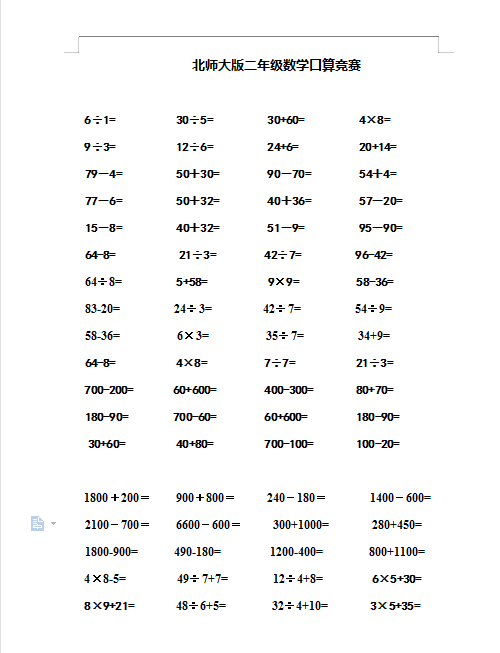6÷1=          30÷5=         30+60=         4×8=

9÷3=          12÷6=         24+6=          20+14=

79－4=    　　 50＋30= 　　   90－70= 　　   54＋4=

77－6= 　　    50＋32= 　　   40＋36= 　  　 57－20=

15－8= 　　    40＋32= 　　   51－9= 　　    95－90=

64-8=             21÷3=        42÷7=          96-42=

2022：新北师大版小学数学2年级下册口算 (9（doc文档1电页子档下载）